# Aldehydes and Ketones

Ketones and aldehydes both contain the carbonyl functional group, $C=O$.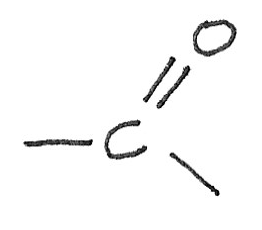The overlap of p-orbital electrons above and below the plane of the carbon and the oxygen atoms result in the formation of a $\pi$ bond. The sideways overlap between the $C$ and $O$ orbitals form a $\sigma$ bond.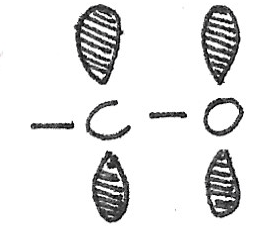### Difference with Alkenes

While alkenes have a double bond between two carbon atoms, the double bond in carbonyls is between a carbon atom and an oxygen atom. In this arrangement, the oxygen atom is more electronegative than a carbon atom. This creates a dipole in the $C=O$ bond as electrons are attracted more to the oxygen atom.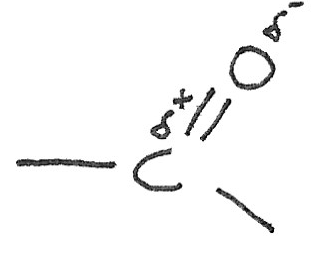#### Naming Aldehydes

In aldehydes the carbonyl functional group is always at the end of the carbon chain. This gives the molecule the -al suffix.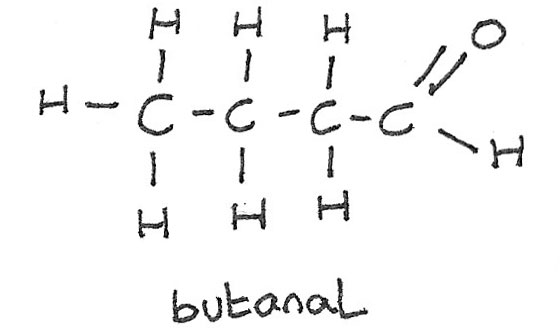#### Naming Ketones

The oxygen atom in ketones can be attached to any of the carbon atoms not at the end of the hydrocarbon chain. Ketones have the -one suffix. It is important to use the smallest possible carbon atom number when naming the molecule.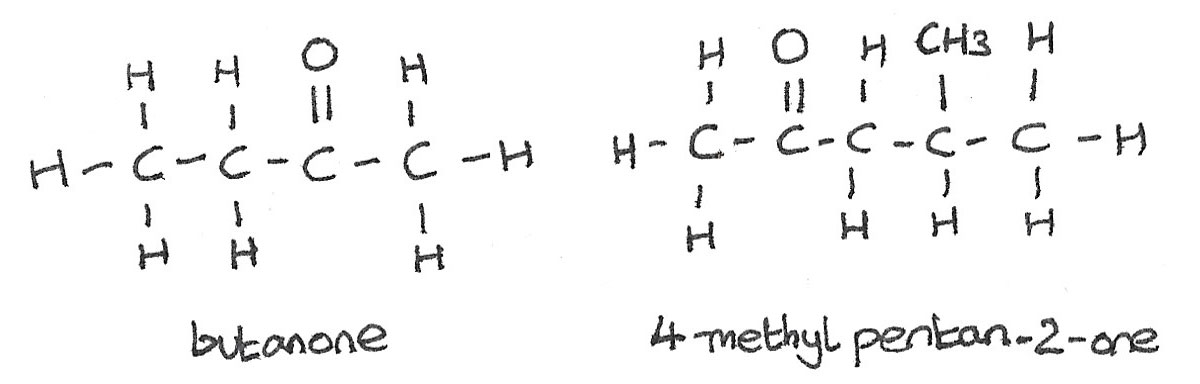## Aromatic Ketones and Aldehydes

Aromatic ketones and aldehydes are compounds that contain both a carbonyl group and a benzene ring. The simplest aromatic aldehyde is benzaldehyde and the simplest aromatic ketone is phenylethanone.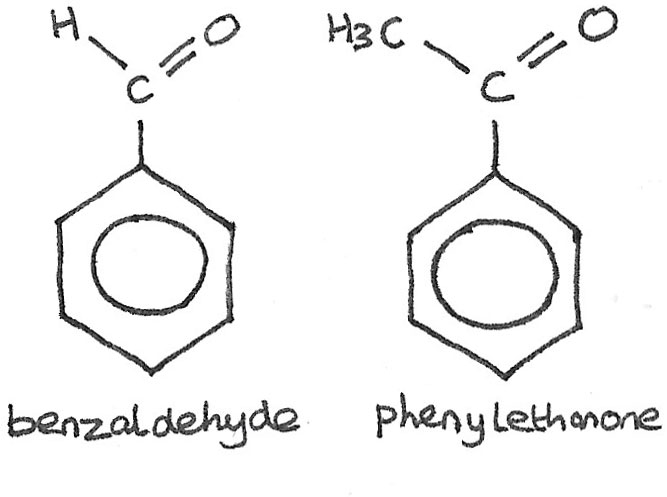## Oxidation of Alcohols

Primary and secondary alcohols can be oxidised using an oxidising agent such as acidified potassium dichromate ($K_{2}Cr_{2}O_{7}$), which supplies the ions $H^{+}/Cr_{2}O_{7}^{~2-}$. Sulphuric acid ($H_{2}SO_{4}$) is used as a catalyst. During the reaction, the solution turns from orange to green.

### Primary Alcohols

These are oxidised first to aldehydes, then oxidised further to carboxylic acids.

$$CH_{3}CH_{2}OH + [O] \rightarrow CH_{3}CHO + H_{2}O \\ CH_{3}CHO + [O] \rightarrow CH_{3}COOH$$

In order to extract an aldehyde, the product must be distilled off as it is produced. To form the carboxylic acid, the reaction mixture must be heated under reflux.

### Secondary Alcohols

Due to the positioning of the $C=O$ bond, secondary alcohols can only be oxidised to form ketones.

$$CH_{3}CHOHCH_{3} + [O] \rightarrow CH_{3}COCH_{3} + H_{2}O$$

### Tertiary Alcohols

Tertiary alcohols are resistant to oxidation. No reaction occurs and therefore the oxidising agent remains orange.

## Reduction of Ketones and Aldehydes

Carbonyl compounds can be reduced to alcohols by warming with a suitable reducing agent such as sodium borohydride ($NaBH_{4}$). Water is often used as the solvent.

#### Aldehydes

Aldehydes are reduced to primary alcohols. In this example, butanal is reduced to butan-1-ol.

$$CH_{3}CH_{2}CH_{2}CHO + 2[H] \rightarrow CH_{3}CH_{2}CH_{2}CH_{2}OH$$

#### Ketones

Ketones are reduced to secondary alcohols. In this example, butanone is reduced to butan-2-ol.

$$CH_{3}CH_{2}COCH_{3} + 2[H] \rightarrow CH_{3}CH_{2}CH(OH)CH_{3}$$

Ketones and aldehydes react with $NaBH_{4}$ in a nucleophilic addition reaction. The $BH_{4}^{-}$ ions acts as a source of the hydride ions, $H^{-}$. These hydride ions act as the nucleophile.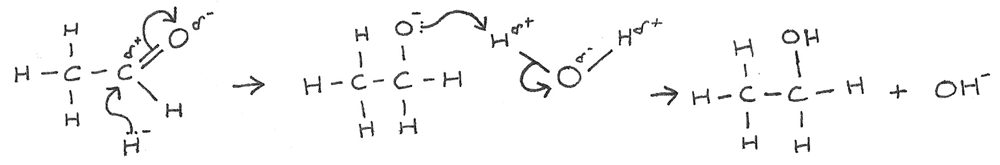1. There is a nucleophilic attack by the hydride ion on the slightly positive carbon atom.
2. The lone pair of electrons from the ${:H^{-}}$ ion forms a dative covalent bond with the carbon atom.
3. At the same time the $\pi$ bond in the $C=O$ bond breaks to produce a negatively charged intermediate.
4. The intermediate then donates an electron pair to a hydrogen atom in $H_{2}O$.
5. This results in the formation of an alcohol and hydroxide ion ($OH^{-}$).

## Chemical Testing of Carbonyl Compounds

Ketones and aldehydes can be detected by using a solution known as Brady's Reagent. Brady's Reagent is a solution of 2,4-dinitrophenylhydrazine ($2,4-DNP$) in a mixture of methanol and sulphuric acid.

Upon adding Brady’s Reagent to an aldehyde or ketone, an orange precipitate is formed, confirming the presence of a $C=O$ bond. This precipitate is known as a 2,4-dinitrophenylhydrazone derivative. There is no visible difference between the reactions between aldehydes and ketones.

#### Further Tests with DNP Derivative

After using Brady’s reagent, a dinitrophenylhydrazone derivative is formed. This solid can be filtered and recrystallised to produce a purified sample. The melting point of this purified product is measured and recorded. The results can be compared to a known database in order to determine the original aldehyde or ketone.

### Tollens' Reagent

Once a compound has been identified as a carbonyl, it can be further identified using Tollens' Reagent. This is a weak oxidising agent that can distinguish between ketones and aldehydes.

Aldehydes are easily oxidised to carboxylic acids, forming a "silver mirror" effect. Ketones and carboxylic acids cannot be oxidised further so no reaction occurs.

Tollens' Reagent can be made by heating aqueous sodium hydroxide with aqueous silver nitrate until a brown precipitate is formed. Dilute aqueous ammonia is added until the precipitate dissolves. This produces Tollens' Reagent, a colourless oxidising agent. The oxidising species is the silver ion, $Ag^{+}_{(aq)}$.

Aldehyde Ketone Carboxylic Acid
Brady's Reagent Orange Precipitate Orange Precipitate No Reaction
Tollens’ Reagent Silver Mirror No Reaction No Reaction